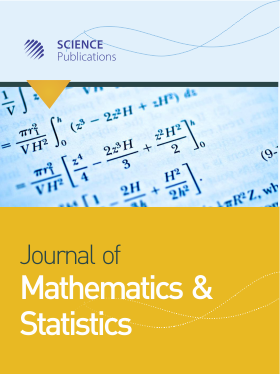Frequency: Continuous
ISSN: 1549-3644 (Print)
ISSN: 1558-6359 (Online)
Research Article Open Access

# Statistical Size and Power of Eight Normality Tests in Presence of GARCH (1 1) Errors

• Julio César Alonso Universidad Icesi, United States

Journal of Mathematics and Statistics
Volume 15 No. 1, 2019, 12-21
DOI:

## Abstract

In this work, we assess the power and size of eight normality tests underthe assumption that errors follow a GARCH (1, 1) process by using MonteCarlo simulations. Four results stand out. First, the presence of a GARCH(1, 1) process increases the probability of making type I error. Second, Pearsonnormality test is recommended if it is assumed that errors follow a GARCH(1, 1) process. Third, statistical power varies depending on the type of heteroscedasticity and distribution considered. Fourth, normality tests have lowstatistical power and size (less than or equal to the nominal level) for smalland homoscedastic samples.

### How to Cite

Alonso, J. C. E. S. & Estrada, D. (2019). Statistical Size and Power of Eight Normality Tests in Presence of GARCH (1 1) Errors. Journal of Mathematics and Statistics, 15(1), 12-21. https://doi.org/10.3844/jmssp.2019.12.21